Next: The magic of sampling Up: Probabilistic Encryption Previous: The Goldwasser-Micali encryption system   Contents

## Weak laws of large numbers

Both the encryption algorithm and the hypothetical algorithms used by the adversary involve random events. We will need a theorem that says that, if an event with probability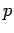is tried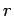times, the chance that the number of successes is not close tois small. The paper uses7(p. 293)

Lemma 24   Letbe the number of successes intries. For any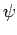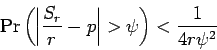Proof:is a random variable, which is the sum ofindependent random variables, each having value 0 or 1. Let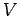be the variance of. Each of the 0-1 variables has variance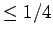, soLemma 24 provides a very rough estimate of the probability. An improvement requiring much more work is:

Lemma 25   With the same notation as Lemma 24,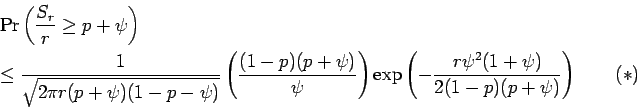For comparison, if,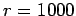, the probability that there aresuccesses is .1087. Lemma 24 gives8an upper limit of .3125, while Lemma 25 gives .1498. (these figures courtesy of Mathematica)

One reason the paper does not use Lemma 25 is that it does not give a simple formula for how largewould have to be in terms of the other quantities. We will not use this result later, and you should skip to section 7.3 unless you like to manipulate formulas.

Proof: We will assumeis integer. From the binomial theorem: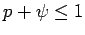implies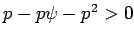andwhich gives the second factor of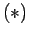. We use Stirling's formula on the binomial coefficient and group it with the powers ofand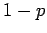to obtain:The first factor ofis the first factor of. We obtain upper bounds on the rest of, using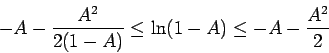(the lower bound on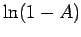involves a geometric series)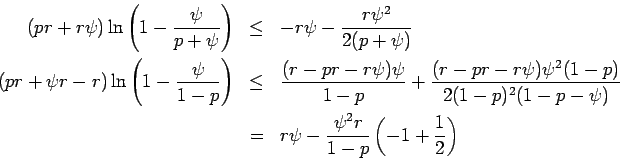Adding these and using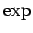gives the remaining factor of.

#### Footnotes

... uses7
The usual central limit theorem cannot be used because it does not tell you how largemust be for the normal distribution to give a good estimate.
... gives8
We divide by 2 to eliminate the probability of.Next: The magic of sampling Up: Probabilistic Encryption Previous: The Goldwasser-Micali encryption system   Contents
Translated from LaTeX by Scott Sutherland
2002-12-14